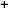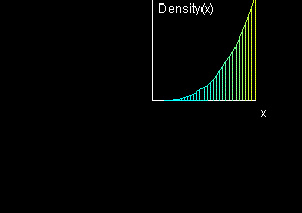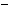Labware - MA35 Multivariable Calculus - Single Variable Calculus
 MA35 Labs 1 » Single Variable Calculus Contents1.4 Integration 1.4.2 Riemann Integral 1.4.5 Change of Variables 1.4.6 Total Mass of an Interval 1.4.7 Center of Mass Search

Total Mass of an Interval

Text

The mass of a one-dimensional object is calculated by integrating its density function over the interval of the domain that it occupies.Demos

 Total Mass of an IntervalThe "Density: rho(x)" window shows the density as a color gradient, where cyan indicates rho=0, yellow rho=1, and red rho=2. The "Mass" window illustrates the mass of the object as the area underneath the function graph of the density function rho.Exercises

• Find the mass of the interval 0 ≤ x ≤ 1 for the density function ρ(x) = x3.
• What is a reasonable constraint on the range of the function ρ(x)?